# 设计模式（二十一）——解释器模式(Spring 框架中SpelExpressionParser源码分析）

## 1 四则运算问题

1) 先输入表达式的形式，比如 a+b+c-d+e,  要求表达式的字母不能重复

2) 在分别输入 a ,b, c, d, e 的值

3) 最后求出结果：如图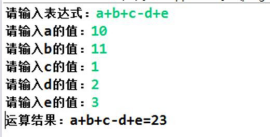## 2 传统方案解决四则运算问题分析

1) 编写一个方法，接收表达式的形式，然后根据用户输入的数值进行解析，得到结果

2) 问题分析：如果加入新的运算符，比如 * / ( 等等，不利于扩展，另外让一个方法来解析会造成程序结构混乱， 不够清晰.

3) 解决方案：可以考虑使用解释器模式  即：  表达式  -> 解释器(可以有多种) -> 结果

## 3 解释器模式基本介绍

1) 在编译原理中，一个算术表达式通过词法分析器形成词法单元，而后这些词法单元再通过语法分析器构建语法分析树，最终形成一颗抽象的语法分析树。这里的词法分析器和语法分析器都可以看做是解释器

2) 解释器模式（Interpreter Pattern）：是指给定一个语言(表达式)，定义它的文法的一种表示，并定义一个解释器， 使用该解释器来解释语言中的句子(表达式)

3) 应用场景

• 应用可以将一个需要解释执行的语言中的句子表示为一个抽象语法树

• 一些重复出现的问题可以用一种简单的语言来表达

• 一个简单语法需要解释的场景

4) 这样的例子还有，比如编译器、运算表达式计算、正则表达式、机器人等

## 4 解释器模式的原理类图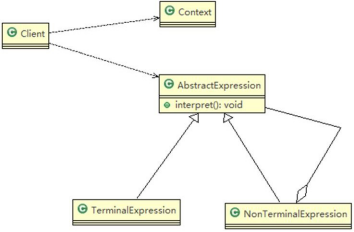• 对原理类图的说明-即(解释器模式的角色及职责)

1) Context: 是环境角色,含有解释器之外的全局信息.

2) AbstractExpression: 抽象表达式， 声明一个抽象的解释操作,这个方法为抽象语法树中所有的节点所共享

3) TerminalExpression: 为终结符表达式, 实现与文法中的终结符相关的解释操作

4) NonTermialExpression: 为非终结符表达式，为文法中的非终结符实现解释操作.

5) 说明： 输入 Context he TerminalExpression  信息通过 Client 输入即可

## 5 解释器模式来实现四则

1) 应用实例要求

2) 思路分析和图解(类图)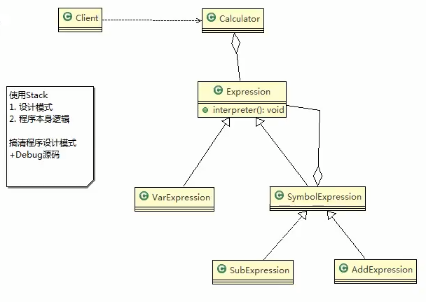3)代码实现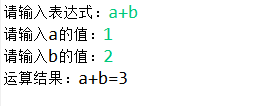```package com.lin.interpret;import java.util.HashMap;    /**
* 抽象类表达式，通过 HashMap 键值对, 可以获取到变量的值
*
* @author Administrator
*     */public abstract class Expression {    // a + b - c    // 解释公式和数值, key  就是公式(表达式) 参数[a,b,c], value 就是就是具体值    // HashMap {a=10, b=20}
public abstract int interpreter(HashMap<String, Integer> var);

}``````package com.lin.interpret;import java.util.HashMap;    /**
* 抽象运算符号解析器 这里，每个运算符号，都只和自己左右两个数字有关系， 但左右两个数字有可能也是一个解析的结果，无论何种类型，都是 Expression
* 类的实现类
*
* @author Administrator
*     */public class SymbolExpression extends Expression {    protected Expression left;    protected Expression right;    public SymbolExpression(Expression left, Expression right) {        this.left = left;        this.right = right;
}    //因为 SymbolExpression  是让其子类来实现，因此 interpreter 是一个默认实现    @Override    public int interpreter(HashMap<String, Integer> var) {        return 0;
}
}``````package com.lin.interpret;import java.util.HashMap;public class SubExpression extends SymbolExpression {    public SubExpression(Expression left, Expression right) {        super(left, right);
}    //求出 left 和 right  表达式相减后的结果
public int interpreter(HashMap<String, Integer> var) {        return super.left.interpreter(var) - super.right.interpreter(var);
}
}``````package com.lin.interpret;import java.util.HashMap;    /**
* 变量的解释器
*
* @author Administrator
*     */public class VarExpression extends Expression {    private String key; // key=a,key=b,key=c

public VarExpression(String key) {        this.key = key;
}    // var 就是{a=10, b=20}    // interpreter 根据 变量名称，返回对应值    @Override    public int interpreter(HashMap<String, Integer> var) {        return var.get(this.key);
}
}``````package com.lin.interpret;import java.util.HashMap;import java.util.Stack;public class Calculator {    // 定义表达式
private Expression expression;    // 构造函数传参，并解析

public Calculator(String expStr) { // expStr = a+b        // 安排运算先后顺序
Stack<Expression> stack = new Stack<>();        // 表达式拆分成字符数组
char[] charArray = expStr.toCharArray();// [a, +, b]
Expression left = null;
Expression right = null;        // 遍历我们的字符数组， 即遍历 [a, +, b]        // 针对不同的情况，做处理
for (int i = 0; i < charArray.length; i++) {            switch (charArray[i]) {            case '+': //                left = stack.pop();// 从 stack 取 出 left => "a"
right = new VarExpression(String.valueOf(charArray[++i]));// 取出右表达式 "b"
stack.push(new AddExpression(left, right));// 然后根据得到 left 和 right 构建 AddExpresson 加入stack
break;            case '-': //                left = stack.pop();
right = new VarExpression(String.valueOf(charArray[++i]));
stack.push(new SubExpression(left, right));                break;            default:                // 如果是一个 Var 就创建要给 VarExpression 对象，并 push 到 stack
stack.push(new VarExpression(String.valueOf(charArray[i])));                break;
}
}        //当遍历完整个 charArray  数组后，stack 就得到最后 Expression
this.expression = stack.pop();
}    public int run(HashMap<String, Integer> var) {    //最后将表达式 a+b 和 var = {a=10,b=20}    //然后传递给 expression 的 interpreter 进行解释执行
return this.expression.interpreter(var);
}
}``````package com.lin.interpret;import java.util.HashMap;    /**
* 加法解释器
*
* @author Administrator
*     */public class AddExpression extends SymbolExpression {    public AddExpression(Expression left, Expression right) {        super(left, right);
}    //处理相加    //var 仍然是 {a=10,b=20}..    //一会我们 debug  源码,就 ok

public int interpreter(HashMap<String, Integer> var) {        //super.left.interpreter(var) ： 返回 left 表达式对应的值 a = 10        //super.right.interpreter(var): 返回 right 表达式对应值 b = 20
return super.left.interpreter(var) + super.right.interpreter(var);
}
}``````package com.lin.interpret;import java.io.BufferedReader;import java.io.IOException;import java.io.InputStreamReader;import java.util.HashMap;public class Client {    public static void main(String[] args) throws IOException {
String expStr = getExpStr(); // a+b
HashMap<String, Integer> var = getValue(expStr);// var {a=10, b=20}
Calculator calculator = new Calculator(expStr);
System.out.println("运算结果：" + expStr + "=" + calculator.run(var));
}    // 获得表达式
public static String getExpStr() throws IOException {
System.out.print("请输入表达式：");        return (new BufferedReader(new InputStreamReader(System.in))).readLine();
}    // 获得值映射
public static HashMap<String, Integer> getValue(String expStr) throws IOException {
HashMap<String, Integer> map = new HashMap<>();        for (char ch : expStr.toCharArray()) {            if (ch != '+' && ch != '-') {                if (!map.containsKey(String.valueOf(ch))) {
System.out.print("请输入" + String.valueOf(ch) + "的值：");
String in = (new BufferedReader(new InputStreamReader(System.in))).readLine();
map.put(String.valueOf(ch), Integer.valueOf(in));
}
}
}        return map;
}

}```## 6 解释器模式在 Spring 框架应用的源码剖析

1) Spring 框架中 SpelExpressionParser 就使用到解释器模式

2) 代码分析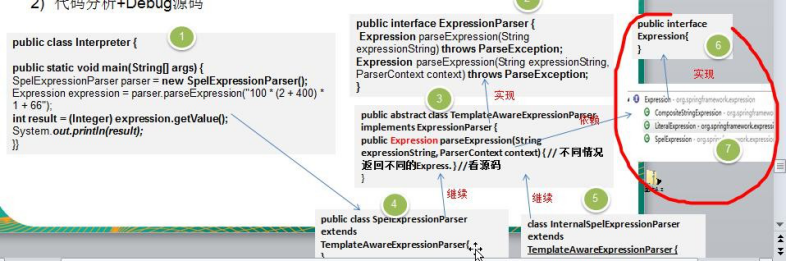3)说明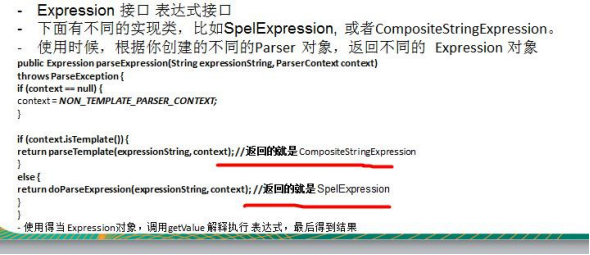## 7 解释器模式的注意事项和细节

1) 当有一个语言需要解释执行，可将该语言中的句子表示为一个抽象语法树，就可以考虑使用解释器模式，让程序具有良好的扩展性

2) 应用场景：编译器、运算表达式计算、正则表达式、机器人等

3) 使用解释器可能带来的问题：解释器模式会引起类膨胀、解释器模式采用递归调用方法，将会导致调试非常复杂、效率可能降低.